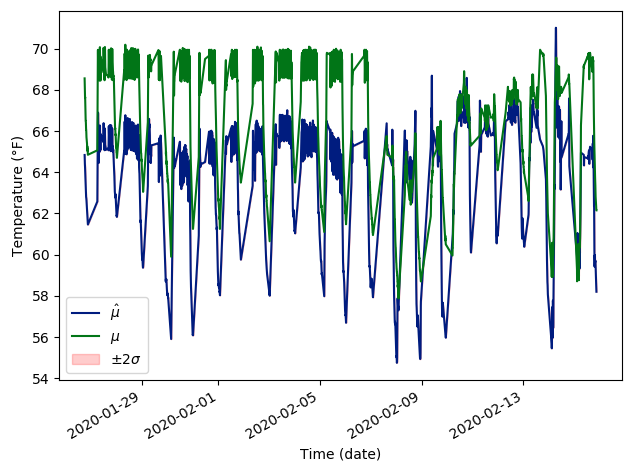# Model with dropout has a bias at test time

I’m training a model for regression, where I’m using an MLP to predict the temperature of a room. In that sense, I’m doing time series forecasting (no RNNs for now), with the addition that not only do I predict the temperature, but also a variance around that prediction. I.e. I’m doing probabilistic forecasting or forecasting with uncertainty estimation, if you will.
Please refer to https://arxiv.org/abs/1612.01474 for more details or even
https://arxiv.org/abs/1506.02142.

I have both approaches implemented in Tensorflow and Pytorch. Originally I observed some strange behavior with Tensorflow that made me re-implement everything in Pytorch (everyone knows Pytorch is better) but it didn’t go away. This behavior is that whenever my model has dropout included in it, at test time, the predictions come with what seems to me to be a bias. Pic attached for clarity.To get the above result, I’m using the Deep Ensembles approach. Some code follows but I can’t share the data.

``````class MLP(nn.Module):

def __init__(self, **kwargs):
super(MLP, self).__init__()
# Activations
self._choices = nn.ModuleDict({
'relu': nn.ReLU(),
'sigmoid': nn.Sigmoid(),
'lrelu': nn.LeakyReLU(),
})

# Initialization parameters
self.num_inputs = kwargs.get('num_inputs', 805)
self.num_outputs = kwargs.get('num_outputs', 1)
self.hidden_size = kwargs.get('hidden_size', 256)
self.activation = kwargs.get('activation', 'relu')

# Optional parameters
self.p = kwargs.get('p', 0.05)

# One hidden layer mlp model
self.model = nn.Sequential(
nn.Linear(self.num_inputs, 2*self.hidden_size),
self._choices[self.activation],
nn.Dropout(self.p),
nn.Linear(2*self.hidden_size, self.hidden_size),
self._choices[self.activation],
nn.Dropout(self.p),
nn.Linear(self.hidden_size, self.num_outputs),
# nn.Dropout(self.p),
)

def forward(self, inputs: torch.Tensor) -> torch.Tensor:
output = self.model(inputs)

return output
``````

And I use this class below

``````class DeepEnsembles(nn.Module):

def __init__(self, **kwargs):
super(DeepEnsembles, self).__init__()

# Algorithm parameters
self.num_models = kwargs.get('num_models')
self.warm_start_it = kwargs.get('warm_start')

# Create models
model = kwargs.get('model')
self.mean = nn.ModuleList([model(**kwargs) for _ in range(self.num_models)])
self.variance = nn.ModuleList([model(**kwargs) for _ in range(self.num_models)])
self.model = nn.ModuleDict({
'mean': self.mean,
'variance': self.variance
})
self._current_it = 0

def predict_with_uncertainty(self, *args, **kwargs) -> Tuple[torch.Tensor, torch.Tensor]:
# Set model to evaluation
self.mean.eval()
self.variance.eval()

# Sample multiple times from the ensemble of models
predictive_mean_list, predictive_variance_list = [], []
for i in range(self.num_models):
predictive_mean = self.mean[i](args)
predictive_mean_list.append(predictive_mean)
predictive_variance = softplus(self.variance[i](args))
predictive_variance_list.append(predictive_variance)

# Stack each of the models
predictive_mean_ensemble = torch.stack(predictive_mean_list)
predictive_variance_ensemble = torch.stack(predictive_variance_list)

# Compute statistics
predictive_mean_model = predictive_mean_ensemble.mean(0)
predictive_variance_model = (predictive_variance_ensemble
+ predictive_mean_ensemble ** 2).mean(0) - predictive_mean_model ** 2
predictive_std_model = predictive_variance_model.sqrt()

return predictive_mean_model, predictive_std_model
``````

Where after training, I simply do

``````mean_predictions_val, variance_predictions_val = algorithm.predict_with_uncertainty(x_val)
``````

This should effectively disable dropout, right? Why am I getting such “biased” predictions at test time? If instead I set `self.mean.train()` and `self.variance.train()` in `predict_with_uncertainty`, I get the following:Which is noisy, but unbiased.

Any thoughts?